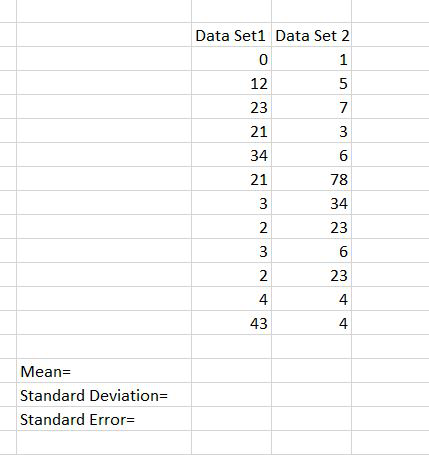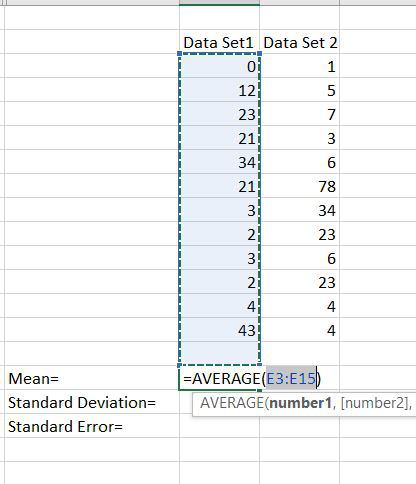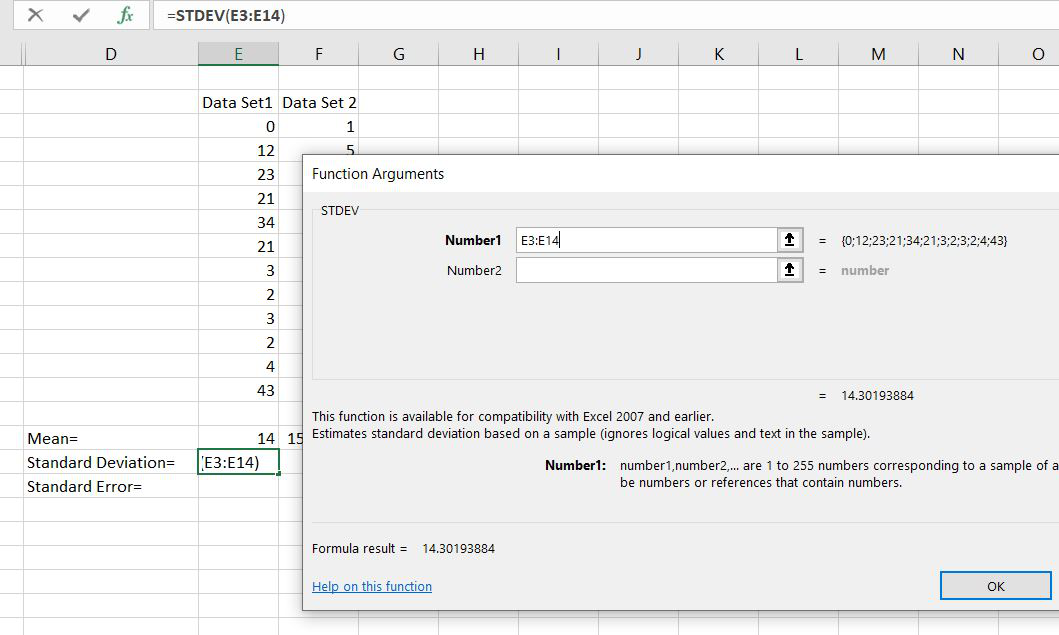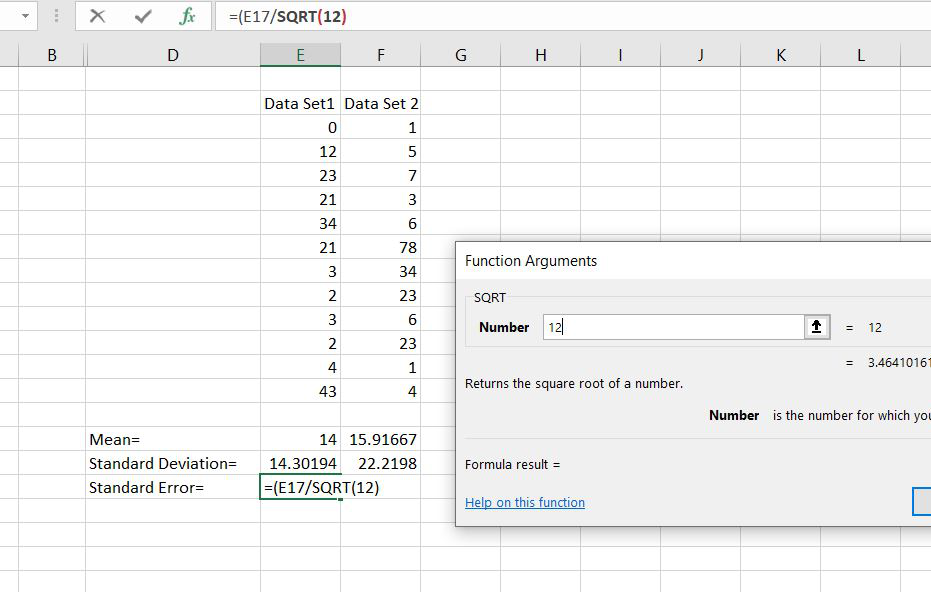Related Articles

# Standard Error in Excel

• Last Updated : 16 Aug, 2021

The standard error tells that how accurate the mean of any given sample from that population is likely to be compared to the true population mean.

Formula:

`Standard Error=Standard Deviation/sqrt(n)`

Where

• n= No. of samples.

Let’s follow the below steps and take a look at an example:

Step 1:  The dataset is given as follow:Step 2:  Now for calculating the standard error we have to find the mean, standard deviation.Step 3: Here we are calculating the standard deviation. And we have selected the rows whose standard deviation we have to calculate.Step 4:  Now for calculating the standard error we have divided the standard deviation with the square root of no. of samples. The no. of samples here are 12.This is the way by which we have calculated the standard error. In this mean was optional.

Attention reader! Don’t stop learning now. If you are an Excel beginner (or an intermediate) and want to learn Excel, Geeksforgeeks brings the perfect course for you to start, Diving Into Excel

My Personal Notes arrow_drop_up